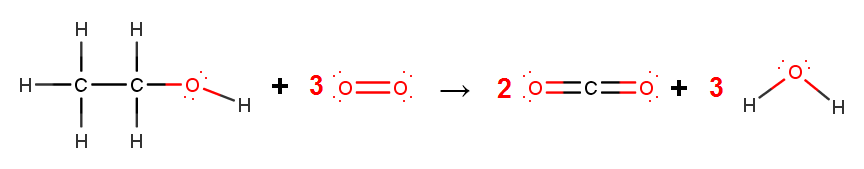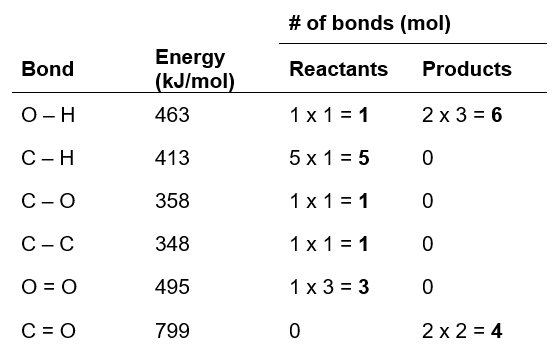# Problem: Ethanol is a possible fuel. Use average bond energies to calculate ΔHrxn for the combustion of ethanol.CH3CH2OH(g) + 3 O2(g) → 2 CO2(g) + 3 H2O(g)

###### FREE Expert Solution

CH3CH2OH(g) + 3 O2(g) → 2 CO2(g) + 3 H2O(g)###### Problem Details

Ethanol is a possible fuel. Use average bond energies to calculate ΔHrxn for the combustion of ethanol.

CH3CH2OH(g) + 3 O2(g) → 2 CO2(g) + 3 H2O(g)